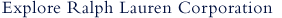# Financial Highlights

## Revenue & Earnings

 Revenue (FY) This is the sum of all revenue (sales) reported for all operating divisions. 6,443.60 EBIT (Mil) (FY) EBIT is computed as Total Revenues for the most recent fiscal year minus Total Operating Expenses plus Operating Interest Expense for the same period. This definition excludes non-operating income and expenses. 770.20 EBIT (Mil) (MRQ) EBIT is computed as Total Revenues for the most recent interim period minus Total Operating Expenses plus Operating Interest Expense for the same period. This definition excludes non-operating income and expenses. 203.80 EBIT (Mil) (TTM) EBIT is computed as Total Revenues for the trailing twelve months minus Total Operating Expenses plus Operating Interest Expense for the same period. This definition excludes non-operating income and expenses. 784.00 EBITDA (Mil) (FY) EBITDA is EBIT for the most recent fiscal year plus the same period's Depreciation and Amortization expenses (from the Statement of Cash Flows). 990.70 EBITDA (Mil) (MRQ) Earnings Before Interest, Taxes, Depreciation and Amortization (EBITDA) is EBIT for the most recent interim period plus the same period's Depreciation and Amortization expenses (from the Statement of Cash Flows). 262.10 EBITDA (Mil) (TTM) Earnings Before Interest, Taxes, Depreciation and Amortization (EBITDA) is EBIT for the trailing twelve months plus the same period's Depreciation and Amortization expenses (from the Statement of Cash Flows) 1,008.00 Net Income Excluding Extraordinary Items (Mil) (FY) Net Income Before Extraordinary Items represents net income before being adjusted by extraordinary items, such as accounting changes, discontinued operations, extraordinary items, and taxes on extraordinary items. 522.70 Net Income Including Extraordinary Items (Mil) (FY) Net Income Including Extraordinary Items represents net income after being adjusted by extraordinary items, such as accounting changes, discontinued operations, extraordinary items, and taxes on extraordinary items. 522.70 Diluted EPS Excluding Extraordinary Items (FY) This is the Adjusted Income Available to Common Stockholders for the most recent fiscal year divided by the most recent fiscal year's Diluted Weighted Average Shares Outstanding. 7.58 Diluted EPS Including Extraordinary Items (FY) This is the Adjusted Income Available to Common Stockholders for the most recent fiscal year plus Discontinued Operations, Extraordinary Items, and Cumulative Effect of Accounting Changes for the same period divided by the most recent fiscal year's Diluted Weighted Average Shares Outstanding. 7.58

## Dividends

 Yield (%) This value is the current percentage dividend yield based on the present cash dividend rate. It is calculated as the Indicated Annual Dividend divided by the current Price, multiplied by 100. 2.61 Dividend Record Date This is the declaration date for latest dividend payment reported by the company. 9/29/23 Dividend Rate This value is the total of the expected dividend payments over the next twelve months. It is generally the most recent cash dividend paid or declared multiplied by the dividend payment frequency, plus any recurring extra dividends. 3.00

## Ratios

 Price to Revenue (TTM) This is the current Price divided by the Sales Per Share for the trailing twelve months. If there is a preliminary earnings announcement for an interim period that has recently ended, the revenue (sales) values from this announcement will be used in calculating the trailing twelve month revenue per share. 1.16 Price to Revenue (MRQ) This is the current Price divided by the Sales Per Share for the most recent interim period. If there is a preliminary earnings announcement for an interim period that has recently ended, the revenue (sales) values from this announcement will be used in calculating the interim Revenues Per Share. 1.25 Price to Revenue (FY) This is the current Price divided by the Sales Per Share for the most recent fiscal year. 1.17 Price To Cash Flow (TTM) This is the current Price divided by Cash Flow Per Share for the trailing twelve months. Cash Flow is defined as Income After Taxes minus Preferred Dividends and General Partner Distributions plus Depreciation, Depletion and Amortization. 9.94 Price to Cash Flow (MRQ) This is the current Price divided by Cash Flow Per Share for the most recent interim period. Cash Flow is defined as Income After Taxes minus Preferred Dividends and General Partner Distributions plus Depreciation, Depletion and Amortization. 9.83 Price to Cash Flow (FY) This is the current Price divided by Cash Flow Per Share for the most recent fiscal year. Cash Flow is defined as Income After Taxes minus Preferred Dividends and General Partner Distributions plus Depreciation, Depletion and Amortization. 10.11 Price to Book (MRQ) This is the Current Price divided by the latest interim period Book Value Per Share. 3.08 Price to Book (FY) This is the Current Price divided by the latest annual Book Value Per Share. 3.11 Total Debt to Equity (MRQ) (%) This ratio is Total Debt for the most recent interim period divided by Total Shareholder Equity for the same period. 60.05% Total Debt to Equity (FY) (%) This ratio is Total Debt for the most recent fiscal year divided by Total Shareholder Equity for the same period. 60.65% Current Ratio (MRQ) This is the ratio of Total Current Assets for the most recent interim period divided by Total Current Liabilities for the same period. 2.18 Current Ratio (FY) This is the ratio of Total Current Assets for the most recent fiscal year divided by Total Current Liabilities for the same period. 2.23

## Growth

 5-Year Annual Dividend Growth Rate (%) This growth rate is the compound annual growth rate of cash dividends per common share of stock over the last 5 years. 8.45% 5-Year Annual Revenue Growth Rate (%) The Five Year Revenue Growth Rate is the annual compounded growth rate of Revenues over the last 5 years. 0.83%

FY = Fiscal Year. MRQ = Most Recent Quarter. mil = Millions. TTM = Trailing Twelve Months.